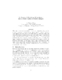## On Number Of Partitions Of An Integer Into A Fixed Number Of Positive IntegersThis paper focuses on the number of partitions of a positive integer $n$ into $k$ positive summands, where $k$ is an integer between $1$ and $n$. Recently some upper bounds were reported for this number in [Merca14]. Here, it is shown that these bounds are not as tight as an earlier upper bound proved in [Andrews76-1] for $k\le 0.42n$. A new upper bound for the number of partitions of $n$ into $k$ summands is given, and shown to be tighter than the upper bound in [Merca14] when $k$ is between $O(\frac{\sqrt{n}}{\ln n})$ and $n-O(\frac{\sqrt{n}}{\ln n})$. It is further shown that the new upper bound is also tighter than two other upper bounds previously reported in~[Andrews76-1] and [Colman82]. A generalization of this upper bound to number of partitions of $n$ into at most $k$ summands is also presented.